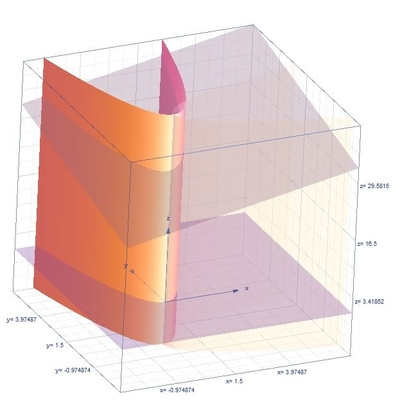# Find the volume of the solid in {eq}\mathrm{R^3} {/eq} bounded by {eq}\; y = x^2, \; x = y^2, \; z = x + y + 30, {/eq} and {eq}\; z = 0 {/eq}.

## Question:

Find the volume of the solid in {eq}\mathrm{R^3} {/eq} bounded by {eq}\; y = x^2, \; x = y^2, \; z = x + y + 30, {/eq} and {eq}\; z = 0 {/eq}.

## Volume of the Region:

The triple integrals formula in rectangular coordinates for the volume of the region is {eq}V=\int \int \int _E dzdydx {/eq}. The limits based on the given equations are the following

{eq}0\leq x\leq 1,\:x^{2}\leq y\leq \sqrt{x},\:0\leq z\leq x+y+30 {/eq}

Become a Study.com member to unlock this answer!

Below is the graph,The volume of the region is,

{eq}V=\int_{0}^{1}\int_{x^{2}}^{\sqrt{x}}\int_{0}^{x+y+30}dzdydx {/eq}

Integrate with...